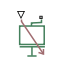# Variable Mass

Time-varying mass specified as physical signal input

## Library

•## Description

This block models the effects of a time-varying mass. Components with variable mass include fuel tanks with progressively lower fuel contents. A physical signal input port provides the instantaneous mass at each time step throughout simulation. To minimize solver errors, avoid discontinuities and abrupt changes in the input signal.

As the component mass changes, so does the inertial force acting on that component:

`${F}_{I}=m\stackrel{˙}{v},$`

where:

• FI is the inertial force.

• m is the variable mass.

• v is the velocity of the mass.

The physical signal input saturates at the minimum mass specified in the block property inspector. Whenever the input falls below the minimum mass, the block performs its calculations on the minimum mass instead. The inertial force becomes:

`${F}_{I}={m}_{Min}\stackrel{˙}{v},$`

where:

• mMin is the minimum mass allowed.

The linear momentum of the variable mass is conserved. In the absence of external forces, a decrease in mass leads to a proportional increase in linear velocity. The linear momentum remains constant until a net external force causes it to change.

### Variables

To set the priority and initial target values for the block variables prior to simulation, use the Initial Targets section in the block dialog box or Property Inspector. For more information, see Set Priority and Initial Target for Block Variables.

Nominal values provide a way to specify the expected magnitude of a variable in a model. Using system scaling based on nominal values increases the simulation robustness. Nominal values can come from different sources, one of which is the Nominal Values section in the block dialog box or Property Inspector. For more information, see Modify Nominal Values for a Block Variable.

## Ports

The block has one conserving translational port and one physical signal input port. The conserving translational port identifies the driveline component whose mass varies with time. The physical signal input port provides the means to specify the mass as a function of time.

## Parameters

Minimum mass

Lowest value that the variable mass can take on during simulation. The physical signal input saturates at this value. If the input falls below the minimum mass, it is set equal to the minimum mass value. The default value is `0.1` `kg`.

## Version History

Introduced in R2015b